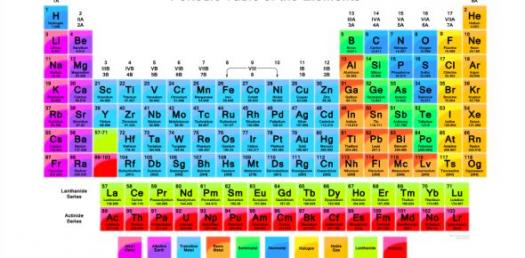# The Periodic Table Unit 4 - Test

17 Questions | Total Attempts: 159SettingsWhat defines and differentiates solids from liquids or gases? Periodic table groups elements according to their atomic volumes. For instance oxygen is a gas and its atomic size is 8. Are you interested in this study of elements and their properties? Try this test on unit 4 to jog your memory.

• 1.
Each energy level of an atom has a maximum number of ____ it can hold.
• A.

Neutrons

• B.

Quarks

• C.

Protons

• D.

Electrons

• 2.
Dot diagrams are used to represent _______.
• A.

The structure of the nucleus

• B.

Atomic number

• C.

Isotopes

• D.

Outer electrons

• 3.
Particles of matter that make up protons and neutrons are _____.
• A.

Electrons

• B.

Isotopes

• C.

Atoms

• D.

Quarks

• 4.
A chemical symbol represents the ____ of an element.
• A.

Name

• B.

Structure

• C.

Reaction

• D.

Type

• 5.
Horizontal rows of the periodic table are called _____.
• A.

Groups

• B.

Families

• C.

Clusters

• D.

Periods

• 6.
Atoms with the same element with different numbers of neutrons are called ____.
• A.

Metalloids

• B.

• C.

Transition elements

• D.

Isotopes

• 7.
A particle that moves around the nucleus is a(n) _____.
• A.

Proton

• B.

Ion

• C.

Neutron

• D.

Electron

• 8.
Elements in groups 3 through 12 are called _______.
• A.

Metalloids

• B.

Transition elements

• C.

Noble gases

• D.

Isotopes

• 9.
A certain atom has 26 protons, 26 electrons, and 30 neutrons. Its mass number is _____.
• A.

26

• B.

30

• C.

52

• D.

56

• 10.
The chart showing the classifications of elements according to their properties and increasing atomic numbers is called the periodic table.
• A.

True

• B.

False

• 11.
Elements arranged in vertical columns in the periodic table are called periods.
• A.

True

• B.

False

• 12.
Two isotopes of carbon are carbon-12 and carbon-14. These isotopes differ from one another by two electrons.
• A.

True

• B.

False

• 13.
Nonmetals are poor conductors of heat and electricity.
• A.

True

• B.

False

• 14.
The sum of the number of protons and the number of neutrons in an atom is the mass number.
• A.

True

• B.

False

• 15.
A diagram showing only outer energy level electrons is called a(n) periodic table.
• A.

True

• B.

False

• 16.
As you move from left to right in a row of the periodic table, metallic properties increase.
• A.

True

• B.

False

Related TopicsBack to top